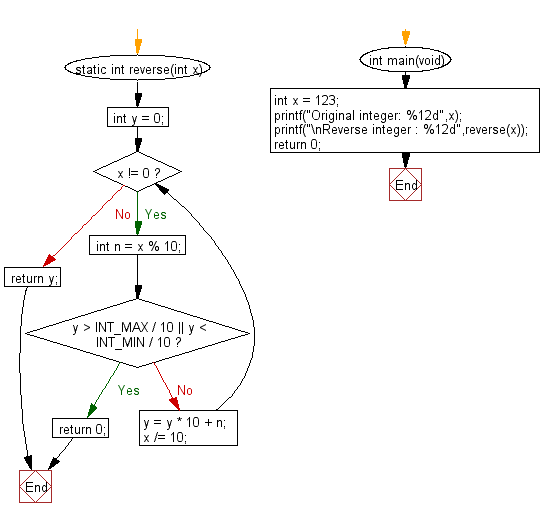﻿ C Program: Reverse digits of a given a 32-bit signed integer - w3resource# C Exercises: Reverse digits of a given a 32-bit signed integer

## C Programming Practice: Exercise-5 with Solution

Write a C programming to reverse digits of a given a 32-bit signed integer.

C Code:

``````#include <stdio.h>
#include <stdlib.h>
#include <limits.h>

static int reverse(int x)
{
int y = 0;
while (x != 0) {
int n = x % 10;
if (y > INT_MAX / 10 || y < INT_MIN / 10) {
return 0;
}
y = y * 10 + n;
x /= 10;
}
return y;
}
int main(void)
{
int x = 123;
printf("Original integer: %12d",x);
printf("\nReverse integer : %12d",reverse(x));
return 0;
}
``````

Sample Output:

```Original integer:          123
Reverse integer :          321
```

Pictorial Presentation:Flowchart:## C Programming Code Editor:

What is the difficulty level of this exercise?

Test your Programming skills with w3resource's quiz.

﻿

## C Programming: Tips of the Day

Maximum value of int:

In C:

```#include <limits.h>
then use
int imin = INT_MIN; // minimum value
int imax = INT_MAX;```

or

```#include <float.h>

float fmin = FLT_MIN;  // minimum positive value
double dmin = DBL_MIN; // minimum positive value

float fmax = FLT_MAX;
double dmax = DBL_MAX;```

Ref : https://bit.ly/3fi8yk9